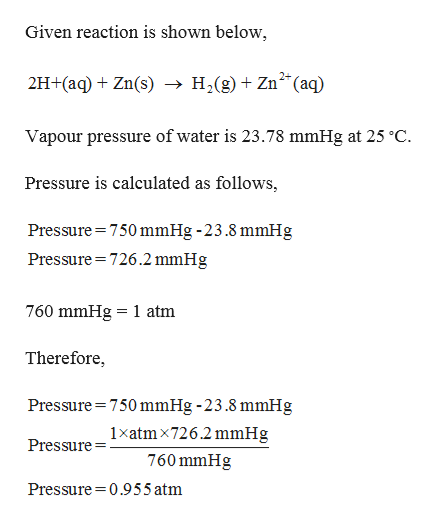# The zinc in a copper-plated penny will dissolve in hydrochloric acid if the copper coating is filed down in several spots (so that the hydrochloric acid can get to the zinc). The reaction between the acid and the zinc is: 2H+(aq)+Zn(s)→H2(g)+Zn2+(aq). When the zinc in a certain penny dissolves, the total volume of gas collected over water at 25 ∘C was 0.957 L at a total pressure of 750 mmHg .What mass of hydrogen gas was collected?

Question
228 views

The zinc in a copper-plated penny will dissolve in hydrochloric acid if the copper coating is filed down in several spots (so that the hydrochloric acid can get to the zinc). The reaction between the acid and the zinc is: 2H+(aq)+Zn(s)→H2(g)+Zn2+(aq).
When the zinc in a certain penny dissolves, the total volume of gas collected over water at 25 ∘C was 0.957 L at a total pressure of 750 mmHg .

What mass of hydrogen gas was collected?

check_circle

Step 1

Given,

Pressure is 750 mmHg.

Litre is 0.957...help_outlineImage TranscriptioncloseGiven reaction is shown below, 2H+(aq)Zn(s) -»H2(g)+Zn (aq) Vapour pressure of water is 23.78 mmHg at 25 °C Pressure is calculated as follows Pressure 750 mmHg -23.8 mmHg Pressure 726.2 mmHg 760 mmHg 1 atm Therefore Pressure 750 mmHg -23.8 mmHg 1xatmx726.2 mmHg Pressure 760 mmHg Pressure 0.955 atm fullscreen

### Want to see the full answer?

See Solution

#### Want to see this answer and more?

Solutions are written by subject experts who are available 24/7. Questions are typically answered within 1 hour.*

See Solution
*Response times may vary by subject and question.
Tagged in

### Chemistry